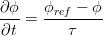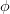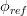# Relaxation$\frac{\partial \phi}{\partial t} = \frac{ \phi_{ref} - \phi}{\tau}$
where$\phi$ is the relaxed variable,$\phi_{ref}$ is the reference solution and$\tau$ relaxation time scale.